Science Journal of Applied Mathematics and Statistics
Volume 4, Issue 3, June 2016, Pages: 97-100

Filtering Analysis of Navigation Data Processing for Personnel Positioning System

Lianhong Ding1, *, Hongqing Sang2, Juntao Li1

1School of Information, Beijing Wuzi University, Beijing, China

2School of Logistics Engineering, School of Information, Beijing Wuzi University, Beijing, China

lhdingbwu@163.com (Lianhong Ding)

*Corresponding author

Lianhong Ding, Hongqing Sang, Juntao Li. Filtering Analysis of Navigation Data Processing for Personnel Positioning System. Science Journal of Applied Mathematics and Statistics. Vol. 4, No. 3, 2016, pp. 97-100. doi: 10.11648/j.sjams.20160403.12

Received: April 18, 2016; Accepted: April 28, 2016; Published: May 13, 2016

Abstract: Ultra wideband technology is a more precise indoor positioning technology. But the UWB positioning output would be unstable if the signal from base station were blocked. The low cost inertial positioning is a method to make up a method for indoor navigation. However, the positioning error will accumulate quickly due to the low cost inertial measurement error. To solve this problem, we selected the MPU6050 module as a chip and Simulated test with Extended Kalman Filter and Unscented Kalman Filter algorithms, and carried out the error analysis on both of them. Finally, come to sampling Kalman filter estimation accuracy estimation is more accurate, more suitable for MPU6050 positioning algorithm.

Keywords: Indoor Positioning, Location Algorithm, Combined Positioning, Extended Calman Filter

Contents

1. Introduction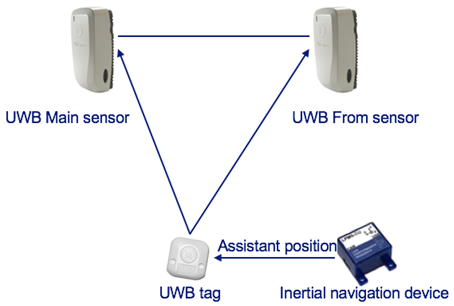Fig. 1. Schematic diagram of auxiliary positioning principle.

Since the indoor environment is more complicated and changeable compare to the outdoor, we must adopt the relatively suitable wireless positioning technology to solve the positioning problem in the indoor environment. To overcome the defects of UWB positioning accuracy, we proposed a method of aided localization of UWB. It combines the user walking state and direction of motion in the position calculation. The positioning system structure is shown in Figure 1. When the UWB positioning signal lost, the inertial guidance sensor records the motion state according to the position of the last position, and carries out the auxiliary positioning of the sensor.

2. Location Algorithm

The positioning data collected by MPU6050 may be noise, so the original data must be filtered to remove the interference data. The emergence of various filtering algorithms provides a theoretical basis and mathematical tools for the processing of navigation data. Filter the basic steps: First, establish the system of state and observation equation; Second, use the appropriate filtering algorithm for optimal system state estimation, filter out the noise part to get the accurate state estimation value. At last, the measurement error of the system is corrected by using the estimated value of these States, so as to obtain the accurate navigation parameter information, so as to achieve the goal of improving the accuracy of the system. The filtering algorithm structure is simple, strong in practicality and widely used in the Extended Kalman Filter and Unscented Kalman Filter algorithms.

3. Extended Kalman Filter

Because the data collected by MPU6050is nonlinear, (EKF)extended Kalman filter is a maximum filtering method for nonlinear state estimation, the basic principle is as a nonlinear model and the of a first-order Taylor series expansion in equation of state estimation value the. Finally, use the traditional Kalman filter to estimate the state.

The state equation and the measurement equation of the nonlinear system are as follows:(1)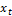,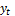are system state estimations; f and h are nonlinear functions;for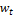the a update number of state noise.

The nonlinear system must be linearized before filtering: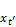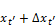(2)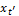for the estimated amount; Δ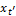is the state to correct the parameters

Taylor series expansion for the state estimation of the Δnonlinear system, and get the approximation: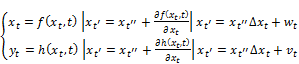(3)

The EKF filtering equation is as follows: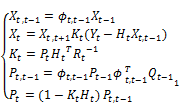(4)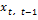is state observation matrix;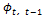is state transition matrix;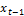is state estimation for t-1 time.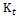is gain matrix;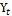is measured value;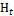is Observation matrix;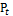is covariance matrix;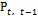is covariance estimation of t-1 time; R is the state of the system; Q is measured variance.

Although EKF can estimate the nonlinear state, it can produce processing errors in the process of linearization: 1. Nonlinear function with the first-order or second-order Taylor, neglecting the higher order terms, the EKF is only suitable for weak nonlinear system, for strong nonlinear system, EKF filtering performance is extremely unstable, and even lead to filter divergence; 2. The complex calculation of Jacobian matrix can be introduced into the linear error estimation, which affects the stability of the system.

Based on the improvement of EKF deficiency, Julier presents a new nonlinear filtering method —— Unscented Kalman Filter. It doesn't linearize to the system equation, but introduces the nonlinear dynamic method with additive noise. UKF uses sigma point sampling to approximate the nonlinear distribution of the system step by step, that is, the combination of the sampling strategy and the Calman filter.

The principle of UT transform is to take some points in the state distribution points according to the X rule. And the covariance and mean of the points are equal to the original state. These points will be used in nonlinear function to obtain the nonlinear function value point set by the strike mean and covariance transformed. In the symmetric sampling, the mean and covariance.

Because the walking state noise and observation noise collected by MPU6050 are assumed as a Gaussian white noise and variance were Q and R, the measuring equation is linear, so there is no need to extend the state dimension, the filtering algorithm is as follows:

Firstly, according to the statistics of the input variable x and the selection of a sigma point sampling strategy, the sigma point set of the input variables and the corresponding mean value W.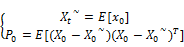(5)

The expected value of the sampling point of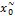for the t=0 time; the covariance matrix of the t=0 time. For time t=1, 2...

A matrix consisting of sigma L points is generated, as shown in the formula (6), according to the principle of the sigma transform, the resulting column vector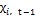.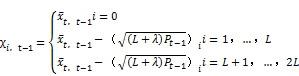(6)

: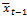is the sample mean of t-1 moment; A is a free parameter;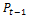is the t-1 time variance; The assumption of discrete nonlinear systems is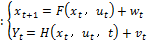, A is the input control vector. The UKF time update equation is: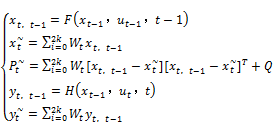(7)is the state observation matrix;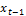is the state estimation of t-1 moment;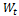is a weight; A is the state predictive value;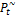is covariance matrix;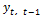is State output matrix;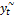is predicted value;

Measurement update equation: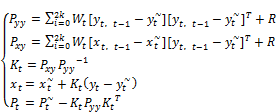(8)

For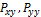in the formula is the predictive variance matrix.

By means of the filter equation, UKF uses a series of sampling points to approximate Gauss distribution, and the recursive and updating of the state and error covariance are carried out by means of UT transform. In each state update process, the sampling point is transferred along with the state equation and the measurement equation is changed. The UKF system can not only ensure the accuracy of state estimation, and because there is no linear transformation, therefore it has better robustness. This is precisely the UKF filter EKF filter is compared superiority.

4. Data Filtering Simulation and Analysis

4.1. MPU6050 Module Data Acquisition

The inertial navigation selected module is MPU6050, selects the UNO Arduino board as the processing monolithic integrated circuit, through the HC-05 Bluetooth module and the upper computer wireless transmission data, as shown in figure 2.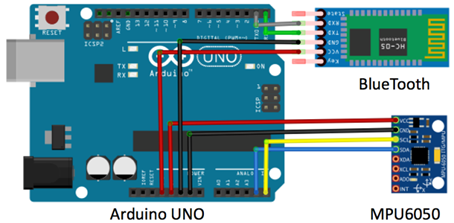Fig. 2. MPU6050 data acquisition module connection diagram.

4.2. Comparative Analysis

There will be some swing because the MPU6050 was worn testers. In addition, because the module of limited precision, so the data will be some errors inevitably. In order to facilitate the observation of curve fitting characteristics, especially the interception of some acceleration curve data of the first 1000ms-1200ms.

As can been seen from Figure 3 and Figure 4, UKF filtering effect is more obvious than EKF jitter is small, and relatively smooth, consistent with the original data curve. Error analysis shown in Figure 5, a progress curve verifies the filtering effect of UKF is better than EKF.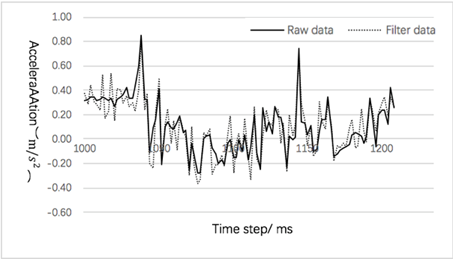Fig. 3. Simulation curves of X axis acceleration for EKF.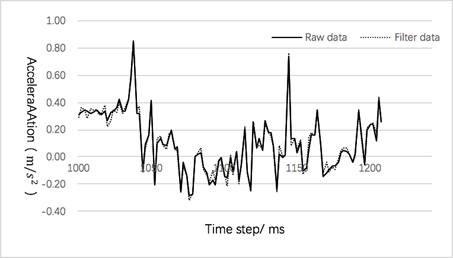Fig. 4. Simulation curves of X axis acceleration for UKF.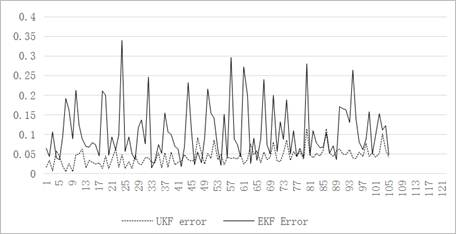Fig. 5. Comparison of EKF and UKF filtering errors.

5. Conclusion and Prospect

EKF linearization method is the system state equation and the measurement equation for local linearization, this method may lead to a suboptimal approximation effect, and may even lead to filter divergence. Using UKF, the state of the nonlinear system model and the error covariance are obtained by using a nonlinear transformation. Based on this collection of testers walking data of extended Kalman filter and nondestructive analysis and Simulation of Kalman filter, as can be seen from Figure 3 and Figure 4 can two kinds of macro filtering can reflect the basic trend of the true curve. While, EKF filtering of signal wobble error is relatively large, there is no actual curve with accurate anastomosis, random error; and UKF overcomes the jitter of the curve well, the curve is much smoother and UKF error is smaller than the EKF, so the UKF has good filtering effect for MPU6050 positioning algorithm which can be seen in Figure 5.

Acknowledgements

This paper is supported by the Funding Project for Technology Key Project of Municipal Education Commission of Beijing (ID:TSJHG201310037036); Funding Project for Beijing key laboratory of intelligent logistics system ;Funding Project of Construction of Innovative Teams and Teacher Career Development for Universities and Colleges Under Beijing Municipality (ID: IDHT20130517), and Beijing Municipal Science and Technology Project (ID: Z131100005413004);Funding Project for Beijing philosophy and social science research base specially commissioned project planning (ID: 13JDJGD013).

References

1. Wang Jianping, Xu Heng, Li Qiyue. The mobile node localization Calman filtering algorithm on [J]. Journal of electronic measurement and instrument based on the 2013, 02: 120-126.
2. Xia Nan, Qiu Tianshuang, Li Jingchun, Li Shufang. A nonlinear filtering algorithm based on Calman filter and particle filter [J]. Journal of electronics, 2013, 01: 148-152.
3. Gao Mingyu. He Zhiwei, Xu Jie. Based on sampling points Kalman filtering power battery SOC estimation [J]. Electrotechnical Journal of, 2011, 11: 161-167.
4. Zhang Qiuzhao, Zhang Shubi, Zheng Nanshan, Wang Jian. The multiple fading robust volume of the combined system of Calman GPS/INS filter [J]. Journal of China University of Mining and Technology, 2014, 01: 162-168.
5. Repair spring wave, Ren Xiao, Li Yanqing, Liu Mingfeng. Short term prediction method of wind speed series based on Calman filter [J]. Journal of electrical engineering, 2014, 02: 253-259.
6. Liu Qi, Yan Li, Zhou. The technical characteristics and development direction of modern telecommunication technology [J]. UWB, 2009, 10: 6-10+18.
7. Wang Hongjian, Jing Wong, Bian Xinqian, Fu Guixia. SLAM [J]. EKF under water robot combined navigation based on 2012, 01: 56-64.
8. Wang Lu, Li Guangchun, Qiao Xiangwei, Wang Zhaolong, Ma Tao. Adaptive UKF algorithm [J]. automation of the maximum likelihood criterion and expectation maximization algorithm based on 2012, 07: 1200-1210.
9. Lin Zhao, Wang Xiaoxu, Sun Ming, Ding Jicheng, Yan Chao. [J]. automation of adaptive UKF filtering algorithm for maximum a posteriori estimation and weighted index based on 2010, 07: 1007-1019.
10. Ge Quanbo, Li Wenbin, Sun Ruoyu, Xu Zi. Estimation of [J]. automation of EKF centralized fusion based on 2013,06:816-825.

 Contents 1. 2. 3. 4. 4.1. 4.2. 5.
Article ToolsAbstractPDF(675K)